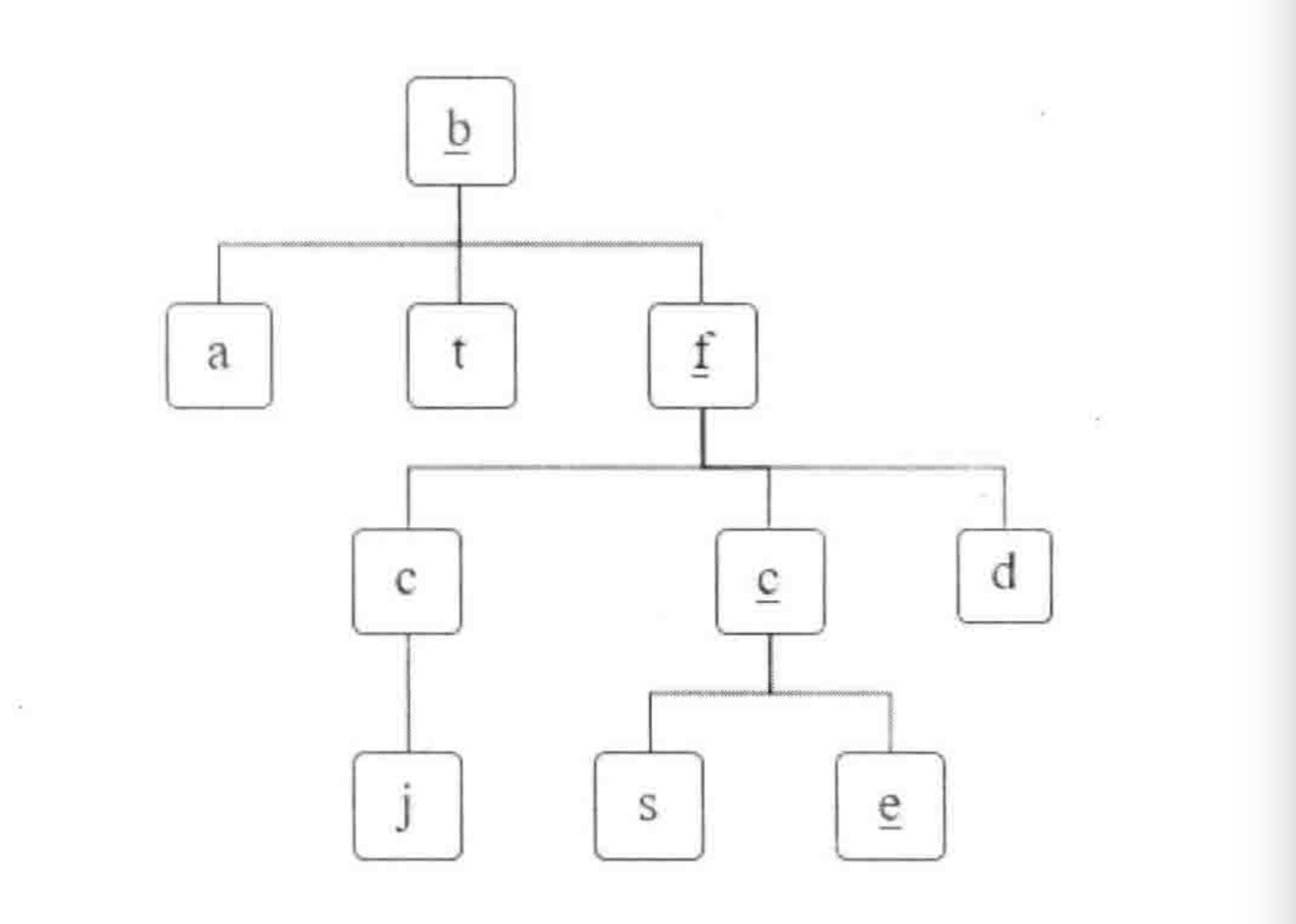## # 题目

``````     a b c e
s f c s
a d e e
``````

## # 思路

• 1.根据给定的行列，遍历字符，根据行列数计算出字符位置
• 2.判断当前字符是否满足递归终止条件
• 3.递归终止条件：(1).行列越界 (2).与路径不匹配 (3).已经走过(需设定一个数组标识当前字符是否走过)
• 4.若路径中的字符最后一位匹配成功，则到达边界且满足约束条件，找到合适的解
• 5.递归不断寻找四个方向是否满足条件，满足条件再忘更深层递归，不满足向上回溯
• 6.如果回溯到最外层，则当前字符匹配失败，将当前字符标记为未走## # 代码

``````    function hasPath(matrix, rows, cols, path) {
const flag = new Array(matrix.length).fill(false);
for (let i = 0; i < rows; i++) {
for (let j = 0; j < cols; j++) {
if (hasPathCore(matrix, i, j, rows, cols, path, flag, 0)) {
return true;
}
}
}
return false;
}

function hasPathCore(matrix, i, j, rows, cols, path, flag, k) {
const index = i * cols + j;
if (i < 0 || j < 0 || i >= rows || j >= cols || matrix[index] != path[k] || flag[index]) {
return false;
}
if (k === path.length - 1) {
return true;
}
flag[index] = true;
if (hasPathCore(matrix, i + 1, j, rows, cols, path, flag, k + 1) ||
hasPathCore(matrix, i - 1, j, rows, cols, path, flag, k + 1) ||
hasPathCore(matrix, i, j + 1, rows, cols, path, flag, k + 1) ||
hasPathCore(matrix, i, j - 1, rows, cols, path, flag, k + 1)) {
return true;
}
flag[index] = false;
return false;
}
``````

• 回溯算法
• 二维数组